Example Questions

Example Question #1 : Acute / Obtuse Triangles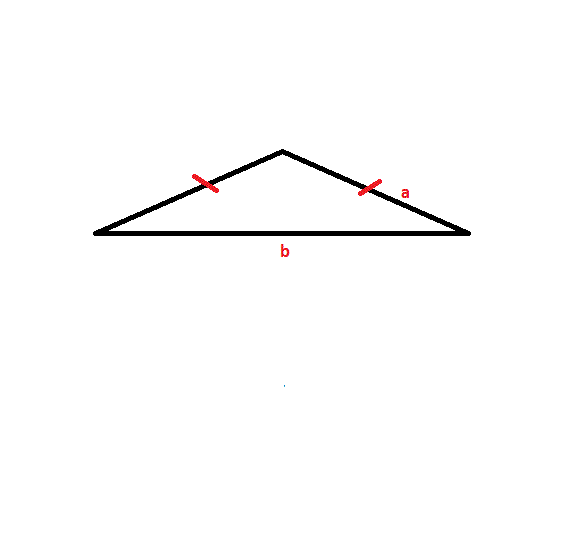The obtuse Isosceles triangle shown above has two sides with lengthand one side length. The length of side. The length of side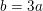. Find the perimeter of the triangle.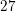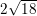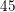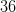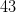Explanation:

To solve this problem, apply the formula: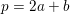Side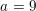, sidemust equal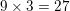Since this is an Isosceles triangle, there must be two sides with a length of.

Thus, plug in each side lengths value to find the solution: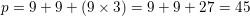Example Question #1 : How To Find An Angle In An Acute / Obtuse Triangle

The three angles in a triangle measure 3x, 4x + 10, and 8x + 20. What is x?

10

30

15

20

25

10

Explanation:

We know the angles in a triangle must add up to 180, so we can solve for x

3x + 4x + 10 + 8x + 20 = 180

15x + 30 = 180

15x = 150

x = 10

Example Question #2 : How To Find An Angle In An Acute / Obtuse Triangle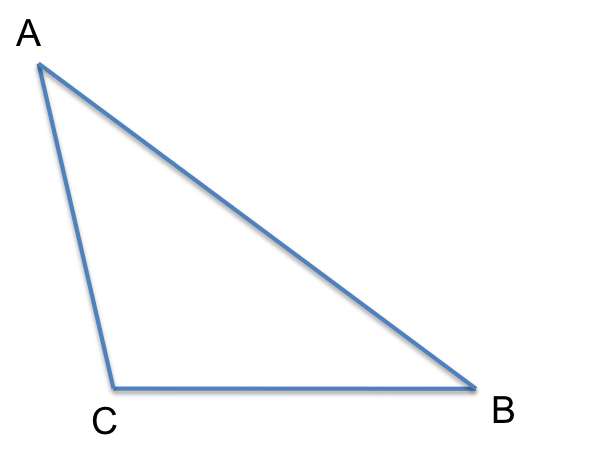In triangle ABC, AB=6, AC=3, and BC=4.

Quantity A                               Quantity B

angle C                   the sum of angle A and angle B

Quantity A is greater

The relationship cannot be determined from the information given.

Quantity A and B are equal

Quantity B is greater

Quantity A is greater

Explanation:

The given triangle is obtuse. Thus, angle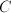is greater than 90 degrees. A triangle has a sum of 180 degrees, so angle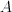+ angle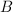+ angle= 180. Since angle C is greater than 90 then angle+ anglemust be less than 90 and it follows that Quantity A is greater.

Example Question #1 : How To Find An Angle In An Acute / Obtuse Triangle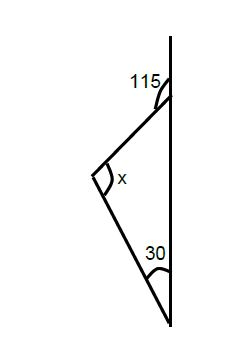In the figure above, what is the value of angle x?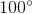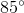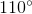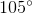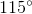Explanation:

To find the top inner angle, recognize that a straight line contains 180o; thus we can subtract 180 – 115 = 65o. Since we are given the other interior angle of 30 degrees, we can add the two we know: 65 + 30 = 95o.

180 - 95 = 85

Example Question #71 : Plane Geometry

What is the area of a triangle with side lengths 18, 24, and 30?

342

280

140

216

196

216

Explanation:

The question doesn't tell us if this is a right triangle, so we can't assume that it is. But there is a formula to find the area when we don't know the height: area = [p(p – a)(p – b)(p – c)]1/2, where a, b, and c are the side lengths and p is half of the perimeter. The perimeter is 18 + 24 + 30 = 72, so p = 72/2 = 36.

Then area = [36(36 – 18)(36 – 24)(36 – 30)]1/2 = [36 * 12 * 6 * 18]1/2 = 216.

Example Question #1261 : Gre Quantitative Reasoning

You are asked which triangle is larger. You are only told that theyhave the same base length and that one contains at least one 3 inch side and the other contains at least one 4 inch side. Determine whether the left or right triangle is larger.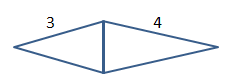It is impossible to determine from the given information.

The triangles are equal

The right triangle is larger

The left triangle is larger

It is impossible to determine from the given information.

Explanation:

Since we are told nothing about the angles we cannot assume that these are isosceles triangles and are open to possibilites such as that shown below in which the left side would be larger. If both were isosceles triangles then the right side would be larger.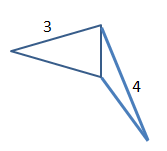Example Question #1 : How To Find The Length Of The Side Of An Acute / Obtuse Triangle

A triangle has sides 3, 5, and x. What can side x not be equal to?

6

9

3

4

9

Explanation:

This question draws from the Third Side Rule of triangles. The length of any side of a triangle must be greater than the difference between the other sides and less than the sum of the other two sides.

This means that side x must be between 2 and 8 since the difference between 5 – 3 = 2 and the sum of 3 + 5 = 8.

Choices 3, 4, and 6 all fall within the range of 2 to 8, but choice 9 does not. The answer is 9.

Example Question #21 : Triangles

Which of these side lengths cannot form a triangle?

7, 7, 12

5, 5, 5

120, 205, 310

25, 37, 66

6, 9, 14

25, 37, 66

Explanation:

Two sides of a triangle must add up to greater than the third side. 25, 37, 66 cannot be the lengths of the sides of a triangle as 25 + 37 < 66.

Example Question #6 : Acute / Obtuse Triangles

The sides of a triangle are 6, 12, and an integer x. How many possible values does x have?

11

1

124

6

2

11

Explanation:

If two sides of a triangle are 6 and 12, the third must be greater than 12-6 and less than 12+6 since two sides cannot be summed to be greater than the third side in a triangle.  There are 11 possible values for x: 7, 8, 9, 10,11, 12, 13, 14, 15, 16, 17.

Example Question #7 : Acute / Obtuse Triangles

Two sides of a triangle are 5 and 7. Which CANNOT be the length of the third side?

9

12

3

5